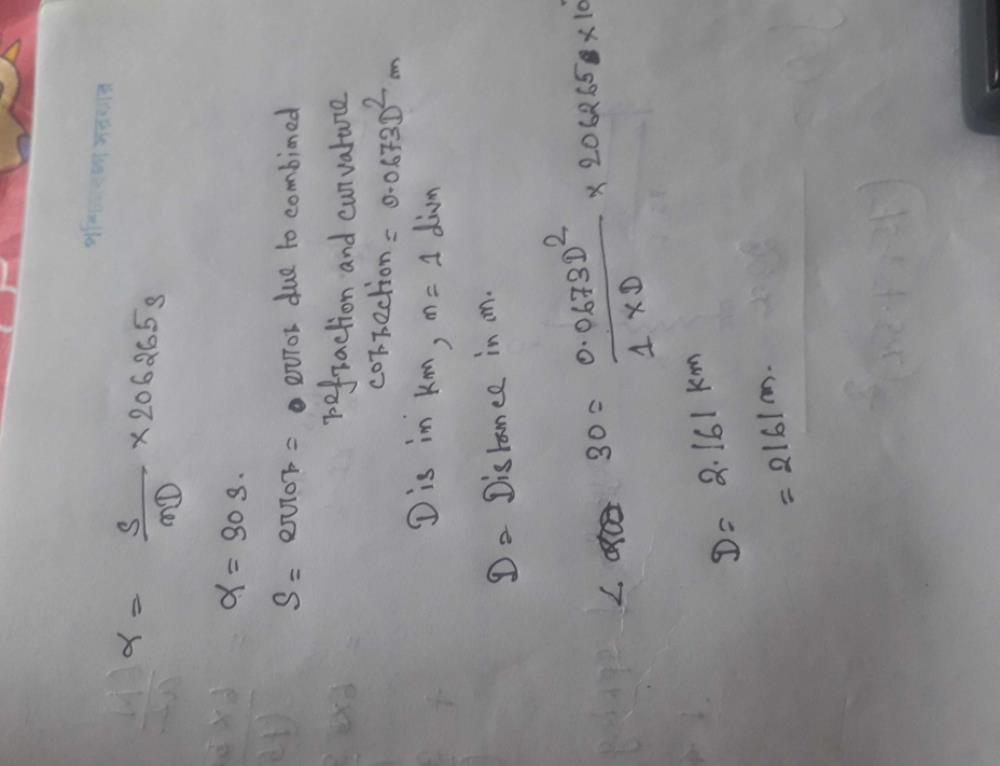Courses

# If the sensitivity of the bubble tube of a level is 30" of arc per division, determine the disn of apoint at which the combined curvature and refraction correction become numerically equalto the error induced by dislevelment of 1 division of the level tube-a)2161.13mb)2522.24mc)2450.62md)2367.26mCorrect answer is option 'A'. Can you explain this answer? Related Test: Technical Mock Test CE (SSC JE)- 14

## Civil Engineering (CE) QuestionS P CHAKRABORTY 3 weeks agoThis discussion on If the sensitivity of the bubble tube of a level is 30 of arc per division, determine the disn of apoint at which the combined curvature and refraction correction become numerically equalto the error induced by dislevelment of 1 division of the level tube-a)2161.13mb)2522.24mc)2450.62md)2367.26mCorrect answer is option 'A'. Can you explain this answer? is done on EduRev Study Group by Civil Engineering (CE) Students. The Questions and Answers of If the sensitivity of the bubble tube of a level is 30 of arc per division, determine the disn of apoint at which the combined curvature and refraction correction become numerically equalto the error induced by dislevelment of 1 division of the level tube-a)2161.13mb)2522.24mc)2450.62md)2367.26mCorrect answer is option 'A'. Can you explain this answer? are solved by group of students and teacher of Civil Engineering (CE), which is also the largest student community of Civil Engineering (CE). If the answer is not available please wait for a while and a community member will probably answer this soon. You can study other questions, MCQs, videos and tests for Civil Engineering (CE) on EduRev and even discuss your questions like If the sensitivity of the bubble tube of a level is 30 of arc per division, determine the disn of apoint at which the combined curvature and refraction correction become numerically equalto the error induced by dislevelment of 1 division of the level tube-a)2161.13mb)2522.24mc)2450.62md)2367.26mCorrect answer is option 'A'. Can you explain this answer? over here on EduRev! Apart from being the largest Civil Engineering (CE) community, EduRev has the largest solved Question bank for Civil Engineering (CE).

### Related Content

This discussion on If the sensitivity of the bubble tube of a level is 30 of arc per division, determine the disn of apoint at which the combined curvature and refraction correction become numerically equalto the error induced by dislevelment of 1 division of the level tube-a)2161.13mb)2522.24mc)2450.62md)2367.26mCorrect answer is option 'A'. Can you explain this answer? is done on EduRev Study Group by Civil Engineering (CE) Students. The Questions and Answers of If the sensitivity of the bubble tube of a level is 30 of arc per division, determine the disn of apoint at which the combined curvature and refraction correction become numerically equalto the error induced by dislevelment of 1 division of the level tube-a)2161.13mb)2522.24mc)2450.62md)2367.26mCorrect answer is option 'A'. Can you explain this answer? are solved by group of students and teacher of Civil Engineering (CE), which is also the largest student community of Civil Engineering (CE). If the answer is not available please wait for a while and a community member will probably answer this soon. You can study other questions, MCQs, videos and tests for Civil Engineering (CE) on EduRev and even discuss your questions like If the sensitivity of the bubble tube of a level is 30 of arc per division, determine the disn of apoint at which the combined curvature and refraction correction become numerically equalto the error induced by dislevelment of 1 division of the level tube-a)2161.13mb)2522.24mc)2450.62md)2367.26mCorrect answer is option 'A'. Can you explain this answer? over here on EduRev! Apart from being the largest Civil Engineering (CE) community, EduRev has the largest solved Question bank for Civil Engineering (CE).